# The physical impossibility of machine computations on sufficiently large integers inspires an open problem that concerns abstract computable sets X⊆N and cannot be formalized in ZFC as it refers to our current knowledge on X

Kurpaska, Sławomir and Tyszka, Apoloniusz (2020) The physical impossibility of machine computations on sufficiently large integers inspires an open problem that concerns abstract computable sets X⊆N and cannot be formalized in ZFC as it refers to our current knowledge on X. [Preprint]There is a more recent version of this item available.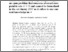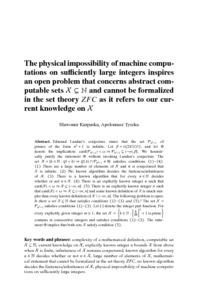Preview
Text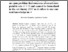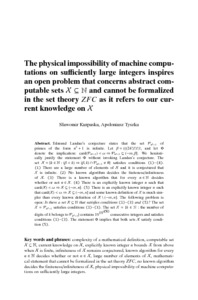Preview
Text
machine_computations.pdf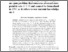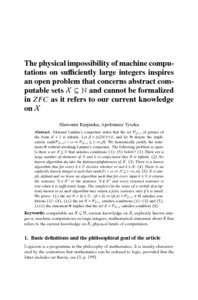Preview
Text
sk_at.pdf

## Abstract

Edmund Landau's conjecture states that the set P(n^2+1) of primes of the form n^2+1 is infinite. Let β=(((24!)!)!)!, and let Φ denote the implication: card(P(n^2+1))<ω ⇒ P(n^2+1)⊆(-∞,β]. We heuristically justify the statement Φ without invoking Landau's conjecture. The following problem is open: Is there a set X⊆N that satisfies conditions (1)-(5) below? (1) There are a large number of elements of X and it is conjectured that X is infinite. (2) No known algorithm decides the finiteness/infiniteness of X . (3) There is a known algorithm that for every k∈N decides whether or not k∈X. (4) There is an explicitly known integer n such that card(X)<ω ⇒ X⊆(-∞,n]. (5) X is simply defined and we know an algorithm such that for every input k∈N it returns the sentence "k∈X" or the sentence "k∉X" and every returned sentence is true when k is sufficiently large. The simplest (in the sense of a verbal description) known to us such algorithm may return a false sentence only if k is small. We prove: (i) the set X ={k∈N: (β<k) ⇒ (β,k)∩P(n^2+1) ≠ ∅} satisfies conditions (1)-(4), (ii) the set X = P(n^2+1) satisfies conditions (1)-(3) and (5,) (iii) the statement Φ implies that the set X= P(n^2+1) satisfies condition (4).

 Export/Citation: EndNote | BibTeX | Dublin Core | ASCII/Text Citation (Chicago) | HTML Citation | OpenURL
 Social Networking:

Item Type: Preprint
Creators:
CreatorsEmailORCID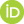Tyszka, Apoloniuszrttyszka@cyf-kr.edu.pl0000-0002-2770-5495
Keywords: computable set X⊆N; current knowledge on X; explicitly known integer n; machine computations on large integers; mathematical statement about X that refers to the current knowledge on X; physical limits of computation
Subjects: Specific Sciences > Mathematics > Foundations
Specific Sciences > Mathematics > Logic
Depositing User: Apoloniusz Tyszka
Date Deposited: 31 Aug 2020 23:21
Item ID: 18027
Subjects: Specific Sciences > Mathematics > Foundations
Specific Sciences > Mathematics > Logic
Date: 16 June 2020
URI: http://philsci-archive.pitt.edu/id/eprint/18027

### Monthly Views for the past 3 yearsView Item